CBSE Class 12 Sample Paper for 2020 Boards

Class 12
Solutions of Sample Papers and Past Year Papers - for Class 12 Boards

## Find the equation of a plane passing through the points A (2, 1, 2) and B (4,  −2, 1) and perpendicular to plane r . (i − 2k) = 5. Also, find the coordinates of the point, where the line passing through the points (3, 4, 1) and (5, 1, 6) crosses the plane thus obtained.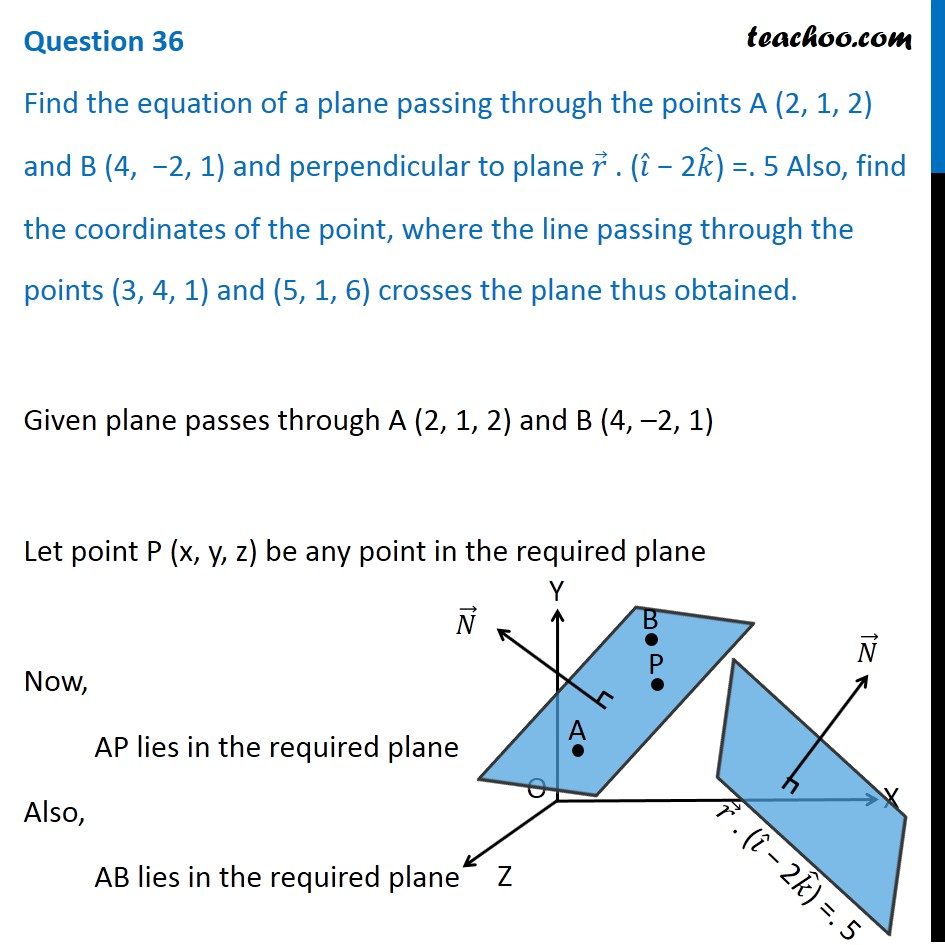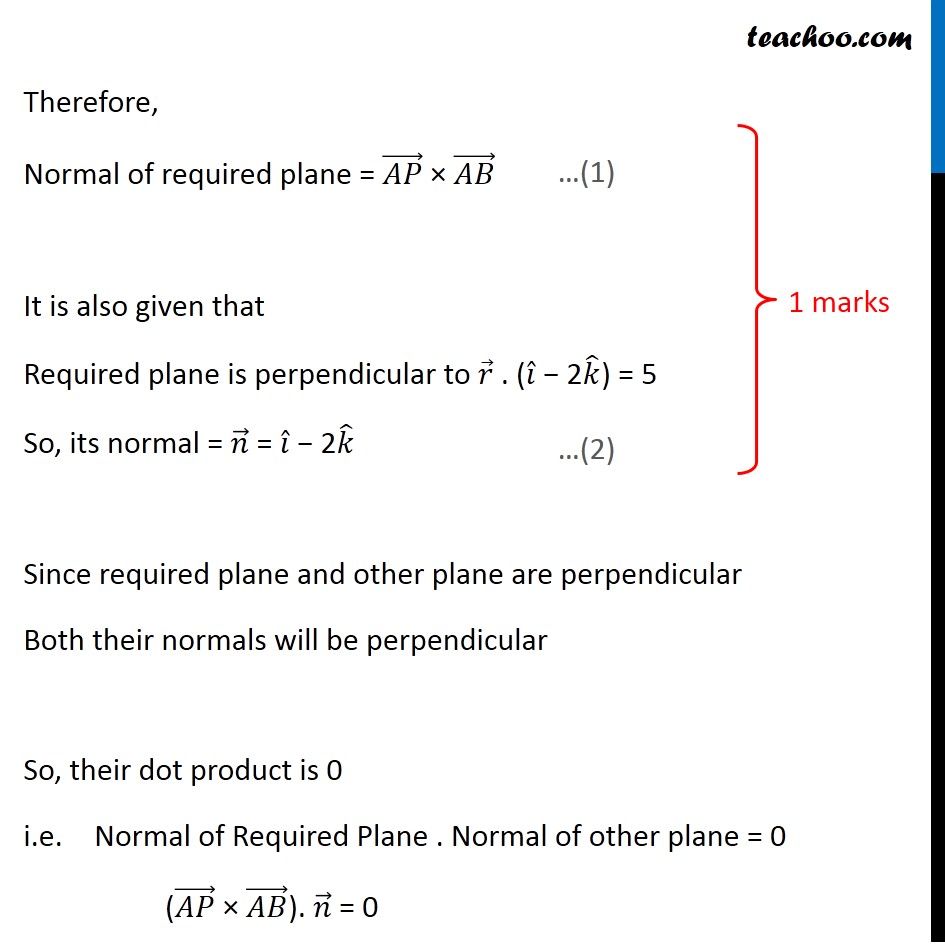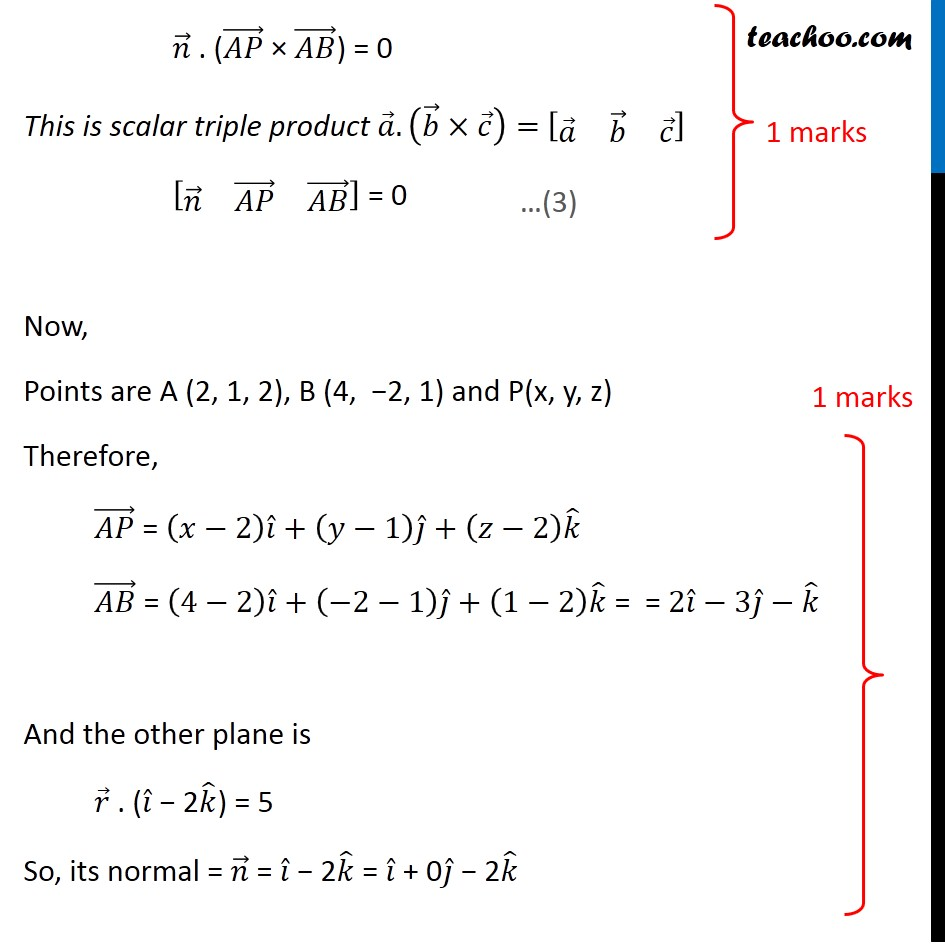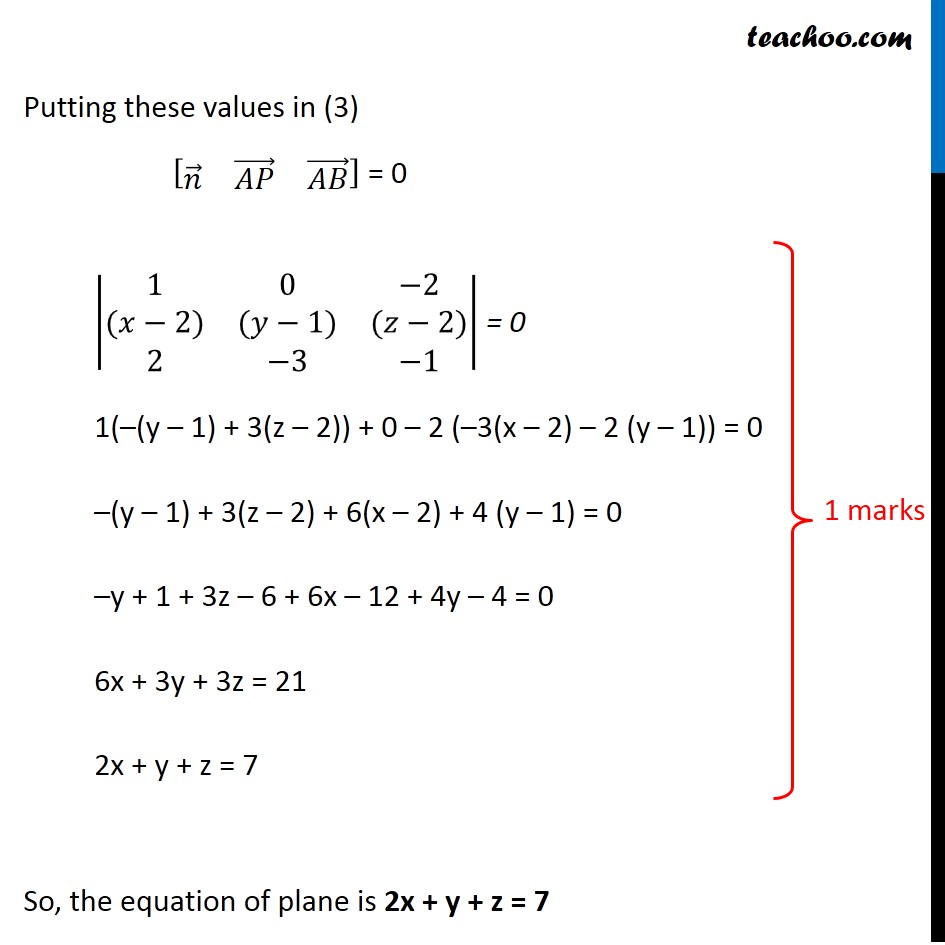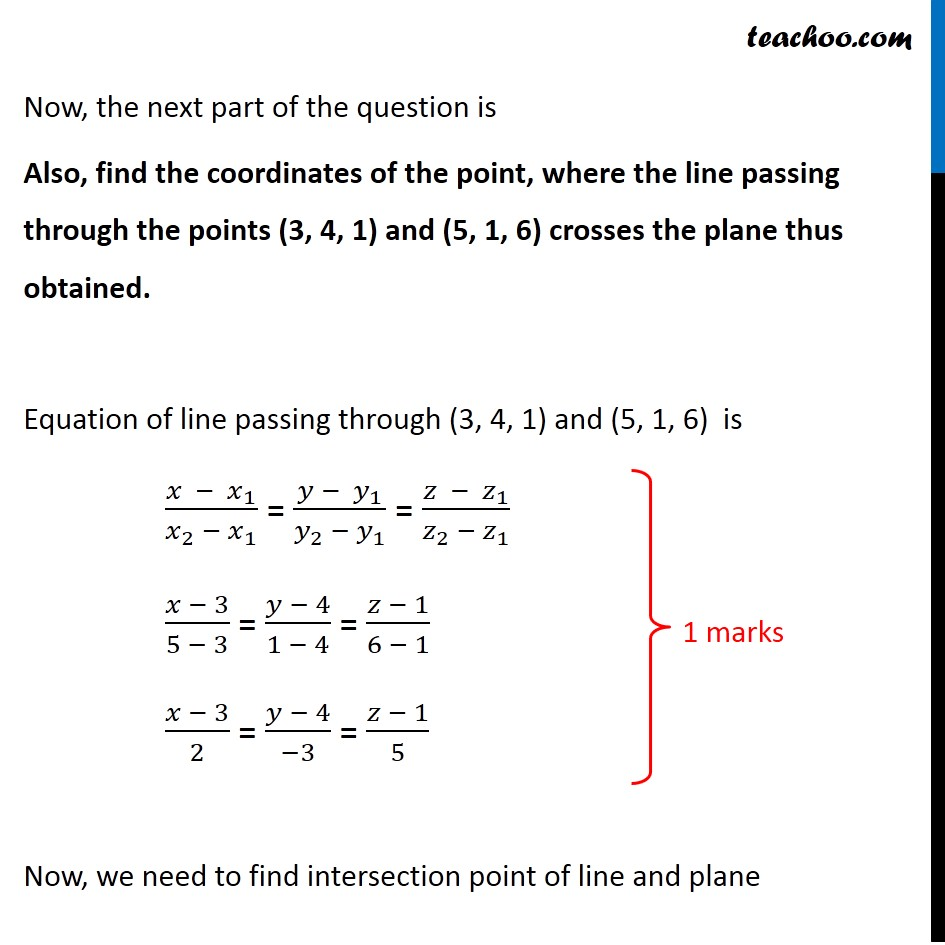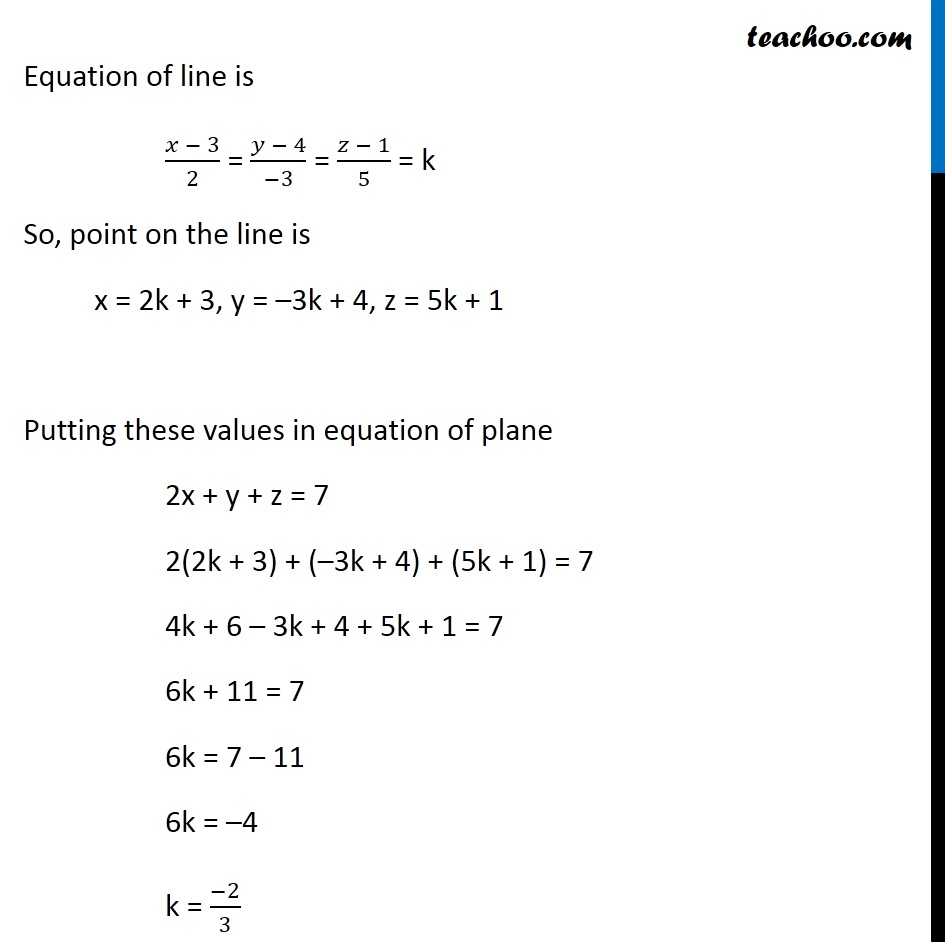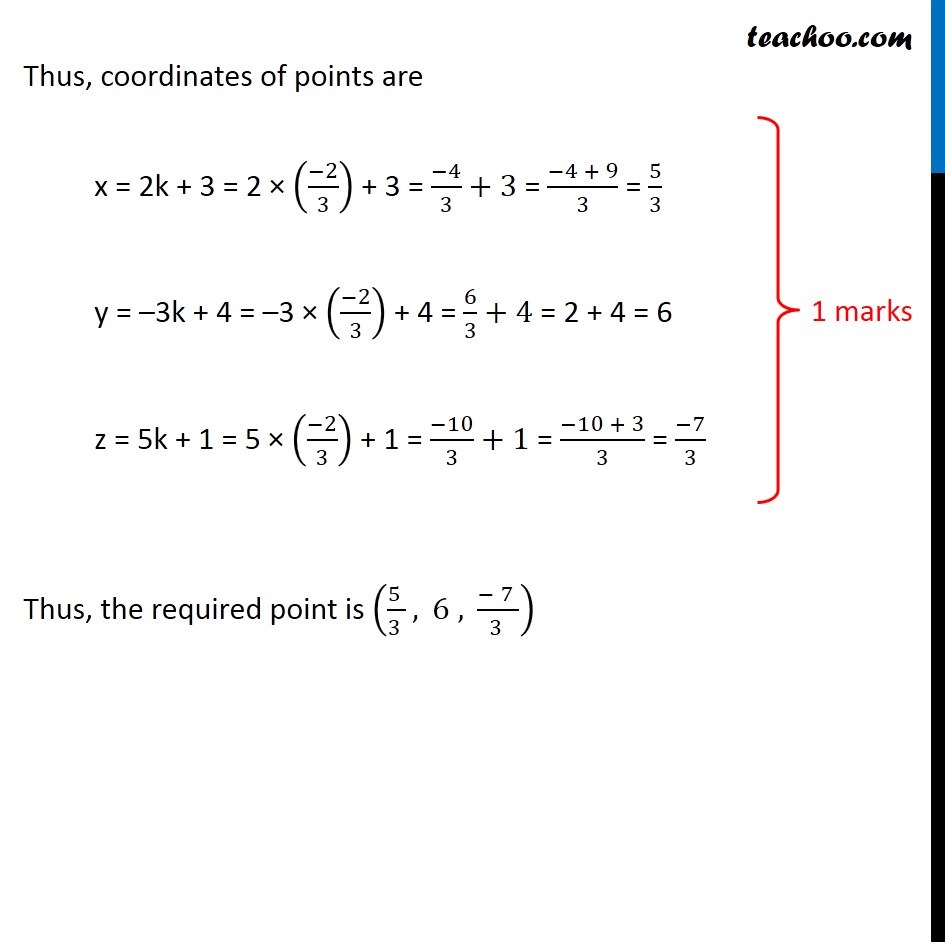Learn in your speed, with individual attention - Teachoo Maths 1-on-1 Class

### Transcript

Question 36 Find the equation of a plane passing through the points A (2, 1, 2) and B (4, −2, 1) and perpendicular to plane 𝑟 ⃗ . (𝑖 ̂ − 2𝑘 ̂) =. 5 Also, find the coordinates of the point, where the line passing through the points (3, 4, 1) and (5, 1, 6) crosses the plane thus obtained. Given plane passes through A (2, 1, 2) and B (4, –2, 1) Let point P (x, y, z) be any point in the required plane Now, AP lies in the required plane Also, AB lies in the required plane Therefore, Normal of required plane = (𝐴𝑃) ⃗ × (𝐴𝐵) ⃗ It is also given that Required plane is perpendicular to 𝑟 ⃗ . (𝑖 ̂ − 2𝑘 ̂) = 5 So, its normal = 𝑛 ⃗ = 𝑖 ̂ − 2𝑘 ̂ Since required plane and other plane are perpendicular Both their normals will be perpendicular So, their dot product is 0 i.e. Normal of Required Plane . Normal of other plane = 0 ((𝐴𝑃) ⃗ × (𝐴𝐵) ⃗). 𝑛 ⃗ = 0 𝑛 ⃗ . ((𝐴𝑃) ⃗ × (𝐴𝐵) ⃗) = 0 This is scalar triple product 𝑎 ⃗.(𝑏 ⃗×𝑐 ⃗ )= [■8(𝑎 ⃗&𝑏 ⃗&𝑐 ⃗ )] [■8(𝑛 ⃗&(𝐴𝑃) ⃗&(𝐴𝐵) ⃗ )] = 0 Now, Points are A (2, 1, 2), B (4, −2, 1) and P(x, y, z) Therefore, (𝐴𝑃) ⃗ = (𝑥−2) 𝑖 ̂+(𝑦−1) 𝑗 ̂+(𝑧−2) 𝑘 ̂ (𝐴𝐵) ⃗ = (4−2) 𝑖 ̂+(−2−1) 𝑗 ̂+(1−2) 𝑘 ̂ = = 2𝑖 ̂−3𝑗 ̂−𝑘 ̂ And the other plane is 𝑟 ⃗ . (𝑖 ̂ − 2𝑘 ̂) = 5 So, its normal = 𝑛 ⃗ = 𝑖 ̂ − 2𝑘 ̂ = 𝑖 ̂ + 0𝑗 ̂ − 2𝑘 ̂ Putting these values in (3) [■8(𝑛 ⃗&(𝐴𝑃) ⃗&(𝐴𝐵) ⃗ )] = 0 |■8(1&0&−2@(𝑥−2)&(𝑦−1)&(𝑧−2)@2&−3&−1)| = 0 1(–(y – 1) + 3(z – 2)) + 0 – 2 (–3(x – 2) – 2 (y – 1)) = 0 –(y – 1) + 3(z – 2) + 6(x – 2) + 4 (y – 1) = 0 –y + 1 + 3z – 6 + 6x – 12 + 4y – 4 = 0 6x + 3y + 3z = 21 2x + y + z = 7 So, the equation of plane is 2x + y + z = 7 Now, the next part of the question is Also, find the coordinates of the point, where the line passing through the points (3, 4, 1) and (5, 1, 6) crosses the plane thus obtained. Equation of line passing through (3, 4, 1) and (5, 1, 6) is (𝑥 − 𝑥_1)/(𝑥_2 − 𝑥_1 ) = (𝑦 − 𝑦_1)/(𝑦_2 − 𝑦_1 ) = (𝑧 − 𝑧_1)/(𝑧_2 − 𝑧_1 ) (𝑥 − 3)/(5 − 3) = (𝑦 − 4)/(1 − 4) = (𝑧 − 1)/(6 − 1) (𝑥 − 3)/2 = (𝑦 − 4)/(−3) = (𝑧 − 1)/5 Now, we need to find intersection point of line and plane Equation of line is (𝑥 − 3)/2 = (𝑦 − 4)/(−3) = (𝑧 − 1)/5 = k So, point on the line is x = 2k + 3, y = –3k + 4, z = 5k + 1 Putting these values in equation of plane 2x + y + z = 7 2(2k + 3) + (–3k + 4) + (5k + 1) = 7 4k + 6 – 3k + 4 + 5k + 1 = 7 6k + 11 = 7 6k = 7 – 11 6k = –4 k = (−2)/3 Thus, coordinates of points are x = 2k + 3 = 2 × ((−2)/3) + 3 = (−4)/3+3 = (−4 + 9)/3 = 5/3 y = –3k + 4 = –3 × ((−2)/3) + 4 = 6/3+4 = 2 + 4 = 6 z = 5k + 1 = 5 × ((−2)/3) + 1 = (−10)/3+1 = (−10 + 3)/3 = (−7)/3 Thus, the required point is (5/3,6,(−7)/3)